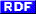# 猫熊は燃えつきた？！日記

2002|12|
2003|01|02|03|04|05|06|07|08|09|10|11|12|
2004|01|02|03|04|05|06|07|08|09|10|11|12|
2005|01|02|03|04|05|06|07|08|09|10|11|12|
2006|01|02|03|04|05|06|07|08|09|10|11|12|
2007|01|02|03|04|05|06|07|08|09|10|11|12|
2008|01|02|03|04|05|06|07|08|09|10|11|12|
2009|01|02|03|04|05|06|07|08|09|10|11|12|
2010|01|02|03|04|05|06|07|08|
2011|09|
2012|02|

## 2005-06-24 (Fri) [長年日記]

### ○ Info で migemo な isearch

CVS Head の Emacs の info.el を参考にしたら、とりあえず動いた。最初、C-r ではじめるとちょっと変だけど気にしない、気にしない。
だけど、これからこういう『賢い isearch』が増えてくるだろうなぁ。ぱぁぁと調べた限りだと hexl が独自の isearch を使っているけど、きちんと対応出来るかなぁ。めちゃくちゃ力づくなんだよな、これ。
```(add-hook 'Info-mode-hook
(lambda ()
(when (and (boundp 'Info-isearch-search)
(featurep 'migemo))
(set (make-local-variable 'isearch-search-fun-function)
'Info-migemo-isearch-search))))

(defun Info-migemo-isearch-search ()
(if Info-isearch-search
(lambda (string &optional bound noerror count)
(cond
(migemo-do-isearch
(Info-migemo-search string bound noerror count
(unless isearch-forward 'backward))
(point))
(isearch-word
(Info-search (concat "\\b" (replace-regexp-in-string
"\\W+" "\\\\W+"
(replace-regexp-in-string
"^\\W+\\|\\W+\$" "" string)) "\\b")
bound noerror count
(unless isearch-forward 'backward)))
(t
(Info-search (if isearch-regexp string (regexp-quote string))
bound noerror count
(unless isearch-forward 'backward))
(point))))
(let ((isearch-search-fun-function nil))
(isearch-search-fun))))

(defun Info-migemo-search (regexp &optional bound noerror count direction)
(when transient-mark-mode
(deactivate-mark))
(let ((backward (eq direction 'backward))
found beg-found give-up
(onode Info-current-node)
(ofile Info-current-file)
(opoint (point))
(opoint-min (point-min))
(opoint-max (point-max))
(ostart (window-start))
(osubfile Info-current-subfile)
(migemo-do-isearch nil))
(setq Info-search-case-fold t)
(save-excursion
(save-restriction
(widen)
(when backward
;; Hide Info file header for backward search
(narrow-to-region (save-excursion
(goto-char (point-min))
(search-forward "\n\^_")
(1- (point)))
(point-max)))
(while (and (not give-up)
(save-match-data
(or (null found)
(if backward
(isearch-range-invisible found beg-found)
(isearch-range-invisible beg-found found))
(and (save-excursion (forward-line -1)
(looking-at "\^_"))
(forward-line (if backward -1 1)))
;; Skip Tag Table node
(save-excursion
(and (search-backward "\^_" nil t)
(looking-at "\^_\nTag Table"))))))
(let (search-spaces-regexp)
(if (if backward
(migemo-backward regexp bound t)
(migemo-forward regexp bound t))
(setq found (point) beg-found (if backward (match-end 0)
(match-beginning 0)))
(setq give-up t))))))

(when (and isearch-mode Info-isearch-search
(not Info-isearch-initial-node)
(not bound)
(or give-up (and found (not (and (> found opoint-min)
(< found opoint-max))))))
(signal 'search-failed (list regexp "initial node")))

;; If no subfiles, give error now.
(if give-up
(if (null Info-current-subfile)
(let (search-spaces-regexp)
(if backward
(migemo-backward regexp)
(migemo-forward regexp)))
(setq found nil)))

(signal 'search-failed (list regexp)))

(unless (or found bound)
(unwind-protect
;; Try other subfiles.
(let ((list ()))
(save-excursion
(set-buffer (marker-buffer Info-tag-table-marker))
(goto-char (point-min))
(search-forward "\n\^_\nIndirect:")
(save-restriction
(narrow-to-region (point)
(progn (search-forward "\n\^_")
(1- (point))))
(goto-char (point-min))
;; Find the subfile we just searched.
(search-forward (concat "\n" osubfile ": "))
;; Skip that one.
(forward-line (if backward 0 1))
(if backward (forward-char -1))
;; Make a list of all following subfiles.
;; Each elt has the form (VIRT-POSITION . SUBFILENAME).
(while (not (if backward (bobp) (eobp)))
(if backward
(re-search-backward "\\(^.*\\): [0-9]+\$")
(re-search-forward "\\(^.*\\): [0-9]+\$"))
(goto-char (+ (match-end 1) 2))
(setq list (cons (cons (+ (point-min)
(match-string-no-properties 1))
list))
(goto-char (if backward
(1- (match-beginning 0))
(1+ (match-end 0)))))
;; Put in forward order
(setq list (nreverse list))))
(while list
(message "Searching subfile %s..." (cdr (car list)))
(when backward
;; Hide Info file header for backward search
(narrow-to-region (save-excursion
(goto-char (point-min))
(search-forward "\n\^_")
(1- (point)))
(point-max))
(goto-char (point-max)))
(setq list (cdr list))
(setq give-up nil found nil)
(while (and (not give-up)
(save-match-data
(or (null found)
(if backward
(isearch-range-invisible found beg-found)
(isearch-range-invisible beg-found found))
(and (save-excursion (forward-line -1)
(looking-at "\^_"))
(forward-line (if backward -1 1)))
;; Skip Tag Table node
(save-excursion
(and (search-backward "\^_" nil t)
(looking-at "\^_\nTag Table"))))))
(let ((search-spaces-regexp Info-search-whitespace-regexp))
(if (if backward
(migemo-backward regexp nil t)
(migemo-forward regexp nil t))
(setq found (point) beg-found (if backward (match-end 0)
(match-beginning 0)))
(setq give-up t))))
(if give-up
(setq found nil))
(if found
(setq list nil)))
(if found
(message "")
(signal 'search-failed (list regexp))))
(goto-char opoint)
(Info-select-node)
(set-window-start (selected-window) ostart)))))

(if (and (string= osubfile Info-current-subfile)
(> found opoint-min)
(< found opoint-max))
;; Search landed in the same node
(goto-char found)
(widen)
(goto-char found)
(save-match-data (Info-select-node)))

;; Use string-equal, not equal, to ignore text props.
(or (and (string-equal onode Info-current-node)
(equal ofile Info-current-file))
(and isearch-mode isearch-wrapped
(eq opoint (if isearch-forward opoint-min opoint-max)))
(setq Info-history (cons (list ofile onode opoint)
Info-history)))))
```

### ○ ダンと夜のお散歩